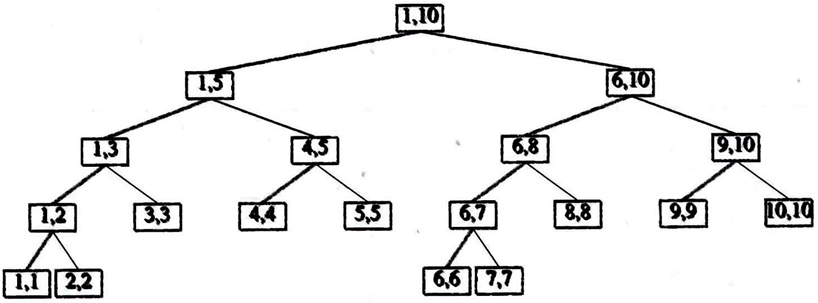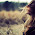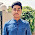## Merge Sort using Java with program code

In computer science, merge sort or mergesort is a sorting algorithm for rearranging lists (or any such linear sequential data storage structure) into a specified order. It is a particularly good example of the divide and conquer algorithmic paradigm. It is a comparison sort. Merging is the process of combining two or more sorted files into a third sorted file.

Conceptually, merge sort works as follows:

1. Divide the unsorted list into two sublists of about half the size
2. Sort each of the two sublists
3. Merge the two sorted sublists back into one sorted list

Merge-Sort Algorithm :

```void MergeSort(int low, int high)
// a[low : high] is a global array to be sorted.
// Small(P) is true if there is only one element to
// sort. In this case the list is already sorted.
{
if (low < high) { // If there are more than one element
// Divide P into subproblems.
// Find where to split the set.
int mid = (low + high)/2;
// Solve the subproblems.
MergeSort(low, mid);
MergeSort(mid + 1, high);
// Combine the solutions.
Merge(low, mid, high);
}
}

void Merge(int low, int mid, int high)
// a[low:high] is a global array containing two sorted
// subsets in a[low:mid] and in a[mid+1:high]. The goal
// is to merge these two sets into a single set residing
// in a[low:high]. b[] is an auxiliary global array.
{
int h = low, i = low, j = mid+1, k;
while ((h <= mid) && (j <= high)) {
if (a[h] <= a[j]) { b[i] = a[h]; h++; }
else { b[i] = a[j]; j++; } i++;
}
if (h > mid) for (k=j; k<=high; k++) {
b[i] = a[k]; i++;
}
else for (k=h; k<=mid; k++) {
b[i] = a[k]; i++;
}
for (k=low; k<=high; k++) a[k] = b[k];
}
```

Example :

a[1:10]=(310, 285, 179, 652, 351, 423, 861, 254, 450, 520)

Hand simulation
(310   285   179   652   351 | 423   861   254   450   520)   split
(310   285   179 | 652   351 | 423   861   254   450   520)   split
(310   285 | 179 | 652   351 | 423   861   254   450   520)   split
(310 | 285 | 179 | 652   351 | 423   861   254   450   520)   split
(285   310 | 179 | 652   351 | 423   861   254   450   520)   merge
(179  285   310  | 652   351 | 423   861   254   450   520)   merge
(179  285   310  | 652 | 351 | 423   861   254   450   520)   split
(179  285   310  | 351   652 | 423   861   254   450   520)   merge
(179  285   310    351   652 | 423   861   254   450   520)   merge
(179  285   310    351   652 | 423   861   254 | 450   520)   split
……

Merge Sort Tree :Advantages :
1. conceptually simple
2. suited to sorting linked lists of elements because merge traverses each linked list
3. suited to sorting external files; divides data into smaller files until can be stored in array in memory
4. stable performance

Two inefficiencies of MergeSort :

1. Not in place (It uses another array b[].)
1.1 Copy between a[] and b[] needed
2. Space and time for stack due to recursion
2.2 For small set sizes, most of time consumed by recursion instead of sorting

Merge Sort Java Code :

```import java.util.*;

public class MergeSort{
public int a[]=new int;
public void merge_sort(int low,int high)
{
int mid;
if(low<high)
{
mid=(low+high)/2;
merge_sort(low,mid);
merge_sort(mid+1,high);
merge(low,mid,high);
}
}
public void merge(int low,int mid,int high)
{
int h,i,j,k;
int b[]=new int;
h=low;
i=low;
j=mid+1;

while((h<=mid)&&(j<=high))
{
if(a[h]<=a[j])
{
b[i]=a[h];
h++;
}
else
{
b[i]=a[j];
j++;
}
i++;
}
if(h>mid)
{
for(k=j;k<=high;k++)
{
b[i]=a[k];
i++;
}
}
else
{
for(k=h;k<=mid;k++)
{
b[i]=a[k];
i++;
}
}
for(k=low;k<=high;k++) a[k]=b[k];
}
public MergeSort()
{
int num,i;

System.out.println("             MERGE SORT PROGRAM            ");

System.out.println();
System.out.println();
System.out.println("Please Enter THE No. OF ELEMENTS you want to sort[THEN PRESS ENTER]:");
num=new Scanner(System.in).nextInt();
System.out.println();
System.out.println("Now, Please Enter the ("+ num +") nos. [THEN PRESS ENTER]:");
for(i=1;i<=num;i++)
{
a[i]=new Scanner(System.in).nextInt() ;
}
merge_sort(1,num);
System.out.println();
System.out.println("So, the sorted list (using MERGE SORT) will be :");
System.out.println();
System.out.println();

for(i=1;i<=num;i++)
System.out.print(a[i]+"    ");

}
public static void main(String[] args) {
new MergeSort();
}
}
```

SHARE THIS POST:

#### 4 comments:

1.Good post Farhan, Merge Sort is a popular sorting mechanism and clear understanding of it helps to understand sorting.

Javin
Multiple ways to Convert String to Integer in Java

2.Thank you for great explanations. Have you done it in-place, since there is no extra array?

3.recurssion at merge-sort is quite confusing how come second mergesort recurssion will ever be called.

4.Thanks. That was a very good explanation.